Wide Supply Range, Rail-to-Rail
Output Instrumentation Amplifier
Rev. 0
Information furnished by Analog Devices is believed to be accurate and reliable. However, no
responsibility is assumed by Analog Devices for its use, nor for any infringements of patents or other
rights of third parties that may result from its use. Specifications subject to change without notice. No
license is granted by implication or otherwise under any patent or patent rights of Analog Devices.
One Technology Way, P.O. Box 9106, Norwood, MA 02062-9106, U.S.A.
Tel: 781.329.4700 www.analog.com
TOP VIEW
(Not to Scale)
07759-001
FEATURES
Gain set with 1 external resistor
Gain range: 5 to 1000
Input voltage goes below ground
Inputs protected beyond supplies
Very wide power supply range
Single supply: 2.2 V to 36 V
Dual supply: ±1.5 V to ±18 V
Bandwidth (G = 5): 250 kHz
CMRR (G = 5): 100 dB minimum (B Grade)
Input noise: 24 nV/√Hz
Typical supply current: 350 μA
Specified temperature: −40°C to +125°C
APPLICATIONS
Industrial process controls
Bridge amplifiers
Medical instrumentation
Portable data acquisition
Multichannel systems
PIN CONFIGURATION
–IN
1
R
G2
R
G3
+IN
4
+V
S
8
V
OUT
7
REF
6
–V
S
5
Figure 1.
Table 1. Instrumentation Amplifiers by Category1
General
Purpose
Zero
Drift
Military
Low
Power
High Speed
PGA
1 See www.analog.com for the latest selection of instrumentation amplifiers.
GENERAL DESCRIPTION
The AD8227 is a low cost, wide supply range instrumentation
amplifier that requires only one external resistor to set any gain
between 5 and 1000.
The AD8227 is designed to work with a variety of signal voltages.
A wide input range and rail-to-rail output allow the signal to
make full use of the supply rails. Because the input range can
also go below the negative supply, small signals near ground can
be amplified without requiring dual supplies. The AD8227
operates on supplies ranging from ±1.5 V to ±18 V (2.2 V to
36 V single supply).
The robust AD8227 inputs are designed to connect to real-
world sensors. In addition to its wide operating range, the
AD8227 can handle voltages beyond the rails. For example,
with a ±5 V supply, the part is guaranteed to withstand ±35 V
at the input with no damage. Minimum as well as maximum
input bias currents are specified to facilitate open wire detection.
The AD8227 is ideal for multichannel, space-constrained
applications. With its MSOP package and 125°C temperature
rating, the AD8227 thrives in tightly packed, zero airflow designs.
The AD8227 is available in 8-pin MSOP and SOIC packages.
It is fully specified for −40°C to +125°C operation.
For a similar instrumentation amplifier with a gain range of 1 toRev. 0 | Page 2 of 24
Features .............................................................................................. 1
Applications ....................................................................................... 1
Pin Configuration ............................................................................. 1
General Description ......................................................................... 1
Revision History ............................................................................... 2
Specifications ..................................................................................... 3
Absolute Maximum Ratings ............................................................ 7
Thermal Resistance ...................................................................... 7
ESD Caution .................................................................................. 7
Pin Configuration and Function Descriptions ............................. 8
Typical Performance Characteristics ............................................. 9
Theory of Operation ...................................................................... 19
Architecture ................................................................................. 19
Gain Selection ............................................................................. 19
Reference Terminal .................................................................... 20
Input Voltage Range ................................................................... 20
Layout .......................................................................................... 20
Input Bias Current Return Path ............................................... 21
Input Protection ......................................................................... 21
Radio Frequency Interference (RFI) ........................................ 21
Applications Information .............................................................. 22
Differential Drive ....................................................................... 22
Precision Strain Gage ................................................................. 22
Outline Dimensions ....................................................................... 24
Ordering Guide .......................................................................... 24
REVISION HISTORY
5/09—Revision 0: Initial VersionRev. 0 | Page 3 of 24
SPECIFICATIONS
+VS = +15 V, −VS = −15 V, VREF = 0 V, TA = 25°C, G = 5, RL = 10 k, specifications referred to input, unless otherwise noted.
Table 2.
Parameter Comments Min Typ Max Min Typ Max Unit
COMMON-MODE REJECTION RATIO VCM = −10 V to +10 V
DC to 60 Hz
G = 5 90 100 dB
G = 10 96 105 dB
G = 100 105 110 dB
G = 1000 105 110 dB
5 kHz
G = 5 80 80 dB
G = 10 86 86 dB
G = 100 86 86 dB
G = 1000 86 86 dB
NOISE Total noise:
eN = √(eNI2 + (eNO/G)2)
Voltage Noise, 1 kHz
Input Voltage Noise, eNI 24 25 24 25 nV/√Hz
Output Voltage Noise, eNO 310 315 310 315 nV/√Hz
RTI f = 0.1 Hz to 10 Hz
G = 5 1.5 1.5 μV p-p
G = 10 0.9 0.9 μV p-p
G = 100 to 1000 0.5 0.5 μV p-p
Current Noise f = 1 kHz 100 100 fA/√Hz
f = 0.1 Hz to 10 Hz 3 3 pA p-p
VOLTAGE OFFSET Total offset voltage:
VOS = VOSI + (VOSO/G)
Input Offset, VOSI V
S = ±5 V to ±15 V 200 100 μV
Average Temperature Drift TA = −40°C to +125°C 0.2 2 0.2 1 μV/°C
Output Offset, VOSO V
S = ±5 V to ±15 V 1000 500 μV
Average Temperature Drift TA = −40°C to +125°C 2 10 2 5 μV/°C
Offset RTI vs. Supply (PSR) VS = ±5 V to ±15 V
G = 5 90 100 dB
G = 10 96 105 dB
G = 100 105 110 dB
G = 1000 105 110 dB
INPUT CURRENT
Input Bias Current1
TA = +25°C 5 20 27 5 20 27 nA
T
A = +125°C 5 15 25 5 15 25 nA
T
A = −40°C 5 30 35 5 30 35 nA
Average Temperature Drift TA = −40°C to +125°C 70 70 pA/°C
Input Offset Current TA = +25°C 1.5 1.5 nA
T
A = +125°C 1.5 1.5 nA
T
A = −40°C 2 2 nA
Average Temperature Drift TA = −40°C to +125°C 5 5 pA/°C
REFERENCE INPUT
RIN 60 60
IIN 12 12 μA
Voltage Range −VS +VS −VS +VS V
Reference Gain to Output 1 1 V/V
Reference Gain Error 0.01 0.01 %Rev. 0 | Page 4 of 24
Parameter Comments Min Typ Max Min Typ Max Unit
DYNAMIC RESPONSE
Small Signal −3 dB Bandwidth
G = 5 250 250 kHz
G = 10 200 200 kHz
G = 100 50 50 kHz
G = 1000 5 5 kHz
Settling Time 0.01% 10 V step
G = 5 14 14 μs
G = 10 15 15 μs
G = 100 35 35 μs
G = 1000 275 275 μs
Slew Rate2
G = 5 to 100 0.8 0.8 V/μs
GAIN3
G = 5 + (80 kΩ/RG)
Gain Range 5 1000 5 1000 V/V
Gain Error VOUT = −10 V to +10 V
G = 5 0.04 0.02 %
G = 10 to 1000 0.3 0.15 %
Gain Nonlinearity VOUT = −10 V to +10 V
G = 5 RL ≥ 2 kΩ 10 10 ppm
G = 10 RL ≥ 2 kΩ 15 15 ppm
G = 100 RL ≥ 2 kΩ 15 50 ppm
G = 1000 RL ≥ 2 kΩ 750 150 ppm
Gain vs. Temperature TA = −40°C to +125°C
G = 5 5 5 ppm/°C
G > 5 −100 −100 ppm/°C
INPUT VS = ±1.5 V to +36 V
Impedance
Differential 0.8||2 0.8||2 GΩ||pF
Common Mode 0.4||2 0.4||2 GΩ||pF
Operating Voltage Range4TA = +25°C −VS − 0.1 +VS − 0.8 −VS − 0.1 +VS − 0.8 V
T
A = +125°C −VS − 0.05 +VS − 0.6 −VS − 0.05 +VS − 0.6 V
T
A = −40°C −VS − 0.15 +VS − 0.9 −VS − 0.15 +VS − 0.9 V
Overvoltage Range TA = −40°C to +125°C +VS − 40 −VS + 40 +VS − 40 −VS + 40 V
OUTPUT
Output Swing
RL = 10 kΩ to ground TA = −40°C to +85°C −VS + 0.2 +VS − 0.2 −VS + 0.2 +VS − 0.2 V
T
A = +85°C to +125°C −VS + 0.2 +VS − 0.3 −VS + 0.2 +VS − 0.3 V
RL = 100 kΩ to ground TA = −40°C to +125°C −VS + 0.1 +VS − 0.1 −VS + 0.1 +VS − 0.1 V
Short-Circuit Current 13 13 mA
POWER SUPPLY
Operating Range Dual-supply operation ±1.5 ±18 ±1.5 ±18 V
Quiescent Current TA = +25°C 350 425 350 425 μA
T
A = −40°C 250 325 250 325 μA
T
A = +85°C 450 525 450 525 μA
T
A = +125°C 525 600 525 600 μA
TEMPERATURE RANGE −40 +125 −40 +125 °C
1 The input stage uses pnp transistors, so input bias current always flows into the part.
2 At high gains, the part is bandwidth limited rather than slew rate limited.
3 For G > 5, gain error specifications do not include the effects of External Resistor RG.
4 Input voltage range of the AD8227 input stage. The input range depends on the common-mode voltage, differential voltage, gain, and reference voltage. See theRev. 0 | Page 5 of 24
+VS = 2.7 V, −VS = 0 V, VREF = 0 V, TA = 25°C, G = 5, RL = 10 k, specifications referred to input, unless otherwise noted.
Table 3.
Parameter Comments Min Typ Max Min Typ Max Unit
COMMON-MODE REJECTION RATIO VCM = 0 V to 1.7 V
DC to 60 Hz
G = 5 90 100 dB
G = 10 96 105 dB
G = 100 105 110 dB
G = 1000 105 110 dB
5 kHz
G = 5 80 80 dB
G = 10 86 86 dB
G = 100 86 86 dB
G = 1000 86 86 dB
NOISE Total noise:
eN = √(eNI2 + (eNO/G)2)
Voltage Noise, 1 kHz
Input Voltage Noise, eNI 25 28 25 28 nV/√Hz
Output Voltage Noise, eNO 310 330 310 330 nV/√Hz
RTI f = 0.1 Hz to 10 Hz
G = 5 1.5 1.5 μV p-p
G = 10 0.8 0.8 μV p-p
G = 100 to 1000 0.5 0.5 μV p-p
Current Noise f = 1 kHz 100 100 fA/√Hz
f = 0.1 Hz to 10 Hz 3 3 pA p-p
VOLTAGE OFFSET Total offset voltage:
VOS = VOSI + (VOSO/G)
Input Offset, VOSI V
S = 0 V to 1.7 V 200 100 μV
Average Temperature Drift TA = −40°C to +125°C 0.2 2 0.2 1 μV/°C
Output Offset, VOSO V
S = 0 V to 1.7 V 1000 500 μV
Average Temperature Drift TA = −40°C to +125°C 2 10 2 5 μV/°C
Offset RTI vs. Supply (PSR) VS = 0 V to 1.7 V
G = 5 90 100 dB
G = 10 96 105 dB
G = 100 105 110 dB
G = 1000 105 110 dB
INPUT CURRENT
Input Bias Current1
TA = +25°C 5 20 27 5 20 27 nA
T
A = +125°C 5 15 25 5 15 25 nA
T
A = −40°C 5 30 35 5 30 35 nA
Average Temperature Drift TA = −40°C to +125°C 70 70 pA/°C
Input Offset Current TA = +25°C 1.5 1.5 nA
T
A = +125°C 1.5 1.5 nA
T
A = −40°C 2 2 nA
Average Temperature Drift TA = −40°C to +125°C 5 5 pA/°C
REFERENCE INPUT
RIN 60 60
IIN 12 12 μA
Voltage Range −VS +VS −VS +VS V
Reference Gain to Output 1 1 V/V
Reference Gain Error 0.01 0.01 %Rev. 0 | Page 6 of 24
Parameter Comments Min Typ Max Min Typ Max Unit
DYNAMIC RESPONSE
Small Signal −3 dB Bandwidth
G = 5 250 250 kHz
G = 10 200 200 kHz
G = 100 50 50 kHz
G = 1000 5 5 kHz
Settling Time 0.01% 2 V step
G = 5 6 6 μs
G = 10 6 6 μs
G = 100 30 30 μs
G = 1000 275 275 μs
Slew Rate2
G = 5 to 10 0.6 0.6 V/μs
GAIN3
G = 5 + (80 kΩ/RG)
Gain Range 5 1000 5 1000 V/V
Gain Error
G = 5 VOUT = 0.8 V to 1.8 V 0.04 0.04 %
G = 10 to 1000 VOUT = 0.2 V to 2.5 V 0.3 0.3 %
Gain vs. Temperature TA = −40°C to +125°C
G = 5 5 5 ppm/°C
G > 5 −100 −100 ppm/°C
INPUT −VS = 0 V; +VS = 2.7 V to
36 V
Impedance
Differential 0.8||2 0.8||2 GΩ||pF
Common Mode 0.4||2 0.4||2 GΩ||pF
Operating Voltage Range4TA = +25°C −0.1 +VS − 0.7 −0.1 +VS − 0.7 V
T
A = −40°C −0.15 +VS − 0.9 −0.15 +VS − 0.9 V
T
A = +125°C −0.05 +VS − 0.6 −0.05 +VS − 0.6 V
Overvoltage Range TA = −40°C to +125°C +VS − 40 −VS + 40 +VS − 40 −VS + 40 V
OUTPUT
Output Swing TA = −40°C to +125°C
RL = 2 kΩ to 1.35 V 0.2 +VS − 0.2 0.2 +VS − 0.2 V
RL = 10 kΩ to 1.35 V 0.1 +VS − 0.1 0.1 +VS − 0.1 V
Short-Circuit Current 13 13 mA
POWER SUPPLY
Operating Range Single-supply operation 2.2 36 2.2 36 V
Quiescent Current +VS = 2.7 V
T
A = +25°C 325 400 325 400 μA
T
A = −40°C 250 325 250 325 μA
T
A = +85°C 425 500 425 500 μA
T
A = +125°C 475 550 475 550 μA
TEMPERATURE RANGE −40 +125 −40 +125 °C
1 Input stage uses pnp transistors, so input bias current always flows into the part.
2 At high gains, the part is bandwidth limited rather than slew rate limited.
3 For G > 5, gain error specifications do not include the effects of External Resistor RG.
4 Input voltage range of the AD8227 input stage. The input range depends on the common-mode voltage, differential voltage, gain, and reference voltage. See the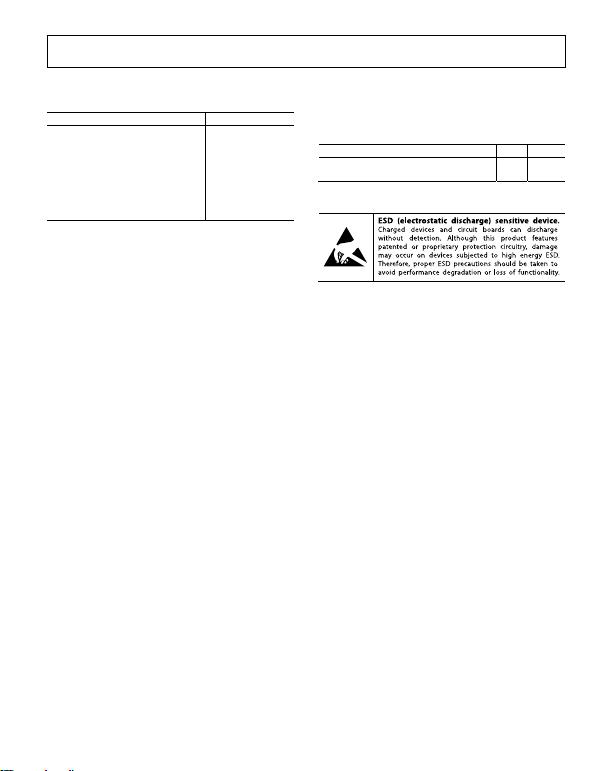Rev. 0 | Page 7 of 24
ABSOLUTE MAXIMUM RATINGS
Table 4.
Parameter Rating
Supply Voltage ±18 V
Output Short-Circuit Current Indefinite
Maximum Voltage at −IN or +IN −VS + 40 V
Minimum Voltage at −IN or +IN +VS − 40 V
REF Voltage ±VS
Storage Temperature Range −65°C to +150°C
Operating Temperature Range −40°C to +125°C
Maximum Junction Temperature 140°C
Stresses above those listed under Absolute Maximum Ratings
may cause permanent damage to the device. This is a stress
rating only; functional operation of the device at these or any
other conditions above those indicated in the operational
section of this specification is not implied. Exposure to absolute
maximum rating conditions for extended periods may affect
device reliability.
THERMAL RESISTANCE
θJA is specified for a device in free air.
Table 5.
Package θJA Unit
8-Lead MSOP, 4-Layer JEDEC Board 135 °C/W
8-Lead SOIC, 4-Layer JEDEC Board 121 °C/W
ESD CAUTIONRev. 0 | Page 8 of 24
TOP VIEW
(Not to Scale)
07759-002
PIN CONFIGURATION AND FUNCTION DESCRIPTIONS
–IN
1
R
G2
R
G3
+V
S
8
V
OUT
7
REF
6
+IN
4
–V
S
5
Figure 2. Pin Configuration
Table 6. Pin Function Descriptions
Pin No. Mnemonic Description
1 −IN Negative Input.
2, 3 RG Gain Setting Pins. Place a gain resistor between these two pins.
4 +IN Positive Input.
5 −VS Negative Supply.
6 REF Reference. This pin must be driven by low impedance.
7 VOUT Output.
8 +VS Positive Supply.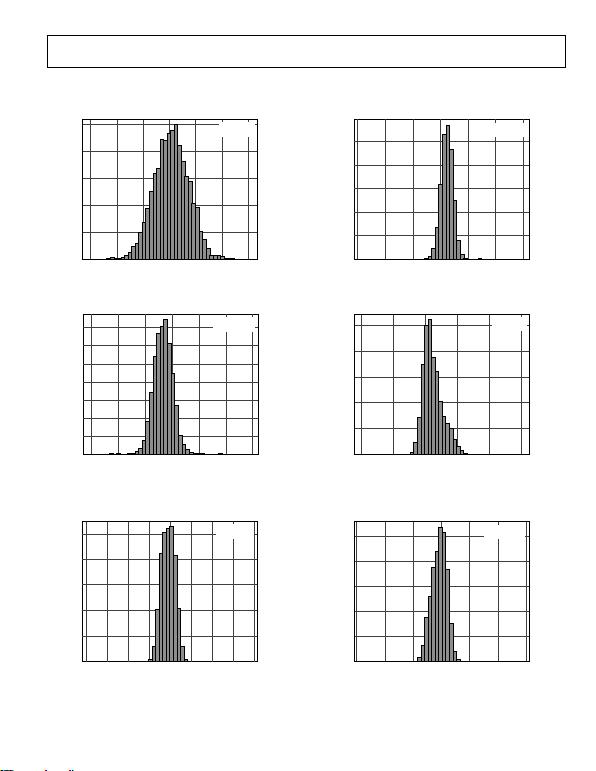Rev. 0 | Page 9 of 24
0
–900 –600 –300 0 300 600 900
OUTPUT V
OS
(µV)
07759-003
TYPICAL PERFORMANCE CHARACTERISTICS
T = 25°C, VS = ±15 V, RL = 10 kΩ, unless otherwise noted.
500
400
300
200
100
HITS
MEAN: 15.9
SD: 196.50
Figure 3. Typical Distribution of Output Offset Voltage
700
600
500
400
300
200
100
HITS
1000
800
600
400
200
0
–0.9 –0.6 –0.3 0 0.3 0.6 0.9
INPUT V
OS
DRIFT (µV)
HITS
07759-006
MEAN: 0.0668
SD: 0.065827
Figure 6. Typical Distribution of Input Offset Voltage Drift, G = 100
0
–6 –4 –2 0 2 4 6
OUTPUT VOS DRIFTV)
07759-004
MEAN: –0.701
SD: 0.676912
Figure 4. Typical Distribution of Output Offset Voltage Drift
1000
800
600
400
200
HITS
1000
800
600
400
200
0
16 18 20 22 24 26
POSITIVE I
BIAS
(nA)
HITS
07759-007
MEAN: 20.4
SD: 0.5893
Figure 7. Typical Distribution of Input Bias Current
0
–200 –150 –100 –50 0 50 100 150 200
INPUT V
OS
(µV)
07759-005
MEAN: –5.90
SD: 15.8825
Figure 5. Typical Distribution of Input Offset Voltage
1000
800
600
400
200
0
–0.9 –0.6 –0.3 0 0.3 0.6 0.9
I
OS
(nA)
HITS
07759-008
MEAN: –0.027
SD: 0.079173
Figure 8. Typical Distribution of Input Offset Current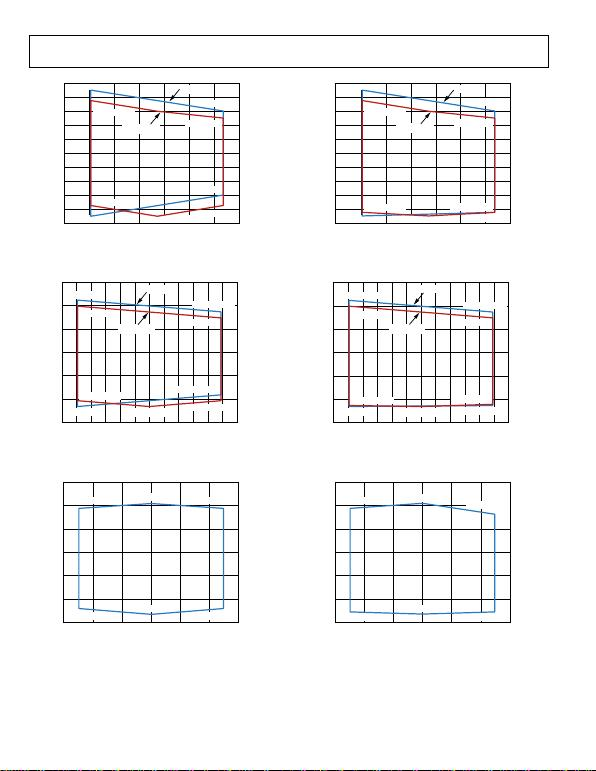Rev. 0 | Page 10 of 24
1.6
1.4
1.2
1.0
0.8
0.6
0.4
0.2
0
–0.2
–0.4
–0.5 0 0.5 1.0 1.5 2.0 2.5 3.0
OUTPUT VOLTAGE (V)
COMMON-MO DE VOLT AGE ( V)
07759-009
+0.0 2V , –0. 3V
+0. 02V, –0 .15V
+0. 02V, + 1.35V
+0.02V, +1.5V V
REF
= 0V
V
REF
= 1. 35V
+1.35V, –0.3V + 2.67V, –0.15V
+2.7V, 0V
+2.67V, +1.2V
+2.7V, +1.1V
Figure 9. Input Common-Mode Voltage vs. Output Voltage,
Single Supply, Vs = 2.7 V, G = 5
5
4
3
2
1
0
–1
–0.5 0 0.5 1.0 1.5 2.0 2.5 3.0 3.5 4.0 4.5 5.0 5.5
OUT P UT VO LTAGE (V)
COMM O N-M O DE VO LT AG E (V )
07759-010
+0. 02V, –0.3V
+0.01 V, –0. 05V
+0.02V, +4V
+0. 02V , +4.25V
+2. 5V, –0.3V +4. 96V , –0.05V
+4.96V, +0.2V
+4.96V, +3.75V
+4.96V, +3.5V
V
REF
= 0V
V
REF
= 2. 5V
Figure 10. Input Common-Mode Voltage vs. Output Voltage,
Single Supply, Vs = 5 V, G = 5
6
4
2
0
–2
–4
–6–6 –4 –2 0 2 4 6
OUTPUT VOLTAGE (V)
COMMON-MODE VOLTAGE (V)
07759-011
–4.98V, +3.7V 0V, +4.2V
0V, –5.3V
–4.97V , –4. 8V
+4.96V, +3.7V
+4. 96V, –4.8V
Figure 11. Input Common-Mode Voltage vs. Output Voltage,
Dual Supply, Vs = ±5 V, G = 5
1.6
1.4
1.2
1.0
0.8
0.6
0.4
0.2
0
–0.2
–0.4
–0.5 0 0.5 1.0 1.5 2.0 2.5 3.0
OUTPUT VOLTAGE (V)
COMMON-MODE VOLTAGE (V)
07759-012
+0. 02V, –0 .25V
+0.02V, +1.35V
+0.02V, +1.5V
+1. 35V, –0.3V
+0.02 V, –0. 3V
+2.67V, – 0.25V
+2. 67V, –0 .25V
+2.67V, +1.2V
+2.67V, +1.1V
V
REF
= 0V
V
REF
= 1. 35V
Figure 12. Input Common-Mode Voltage vs. Output Voltage,
Single Supply, Vs = 2.7 V, G = 100
5
4
3
2
1
0
–1
–0.5 0 0.5 1.0 1.5 2.0 2.5 3.0 3.5 4.0 4.5 5.0 5.5
OUTPUT VOLTAGE (V)
COMMON-MODE VOLTAGE (V)
07759-013
+0.02V, +4.25V
+0.02V, +4V +4.96V, +3.75V
+4.96V, +3.5V
+4. 96V , –0. 25V
+4.9 6V, –0. 2V
+0.02V, –0.3V
+0. 02V , –0. 25V
+2. 5V , –0. 3V
V
REF
= 0V
V
REF
= 2. 5V
Figure 13. Input Common-Mode Voltage vs. Output Voltage,
Single Supply, Vs = 5 V, G = 100
6
4
2
0
–2
–4
–66420246
OUTPUT VOLTAGE (V)
COMMON-MODE VOLTAGE (V)
07759-014
–4.96V , +3.75V 0V, +4.2V
0V, –5.3V
–4.96V , –5. 1V
+4. 96V, + 3.25V
+4.96V, –5.1V
Figure 14. Input Common-Mode Voltage vs. Output Voltage,
Dual Supply, Vs = ±5 V, G = 100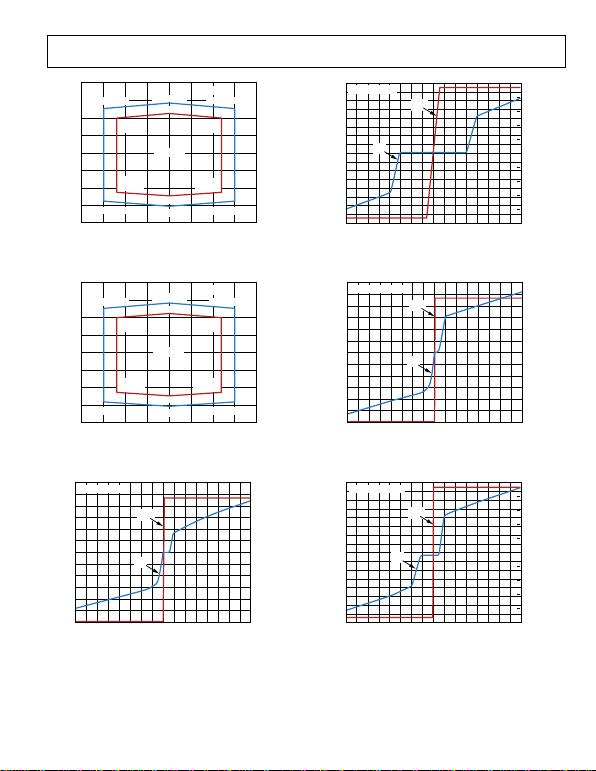Rev. 0 | Page 11
20
15
10
5
0
–5
–10
–15
–20
–20 –15 –10 –5 0 5 10 15 20
COMMON-MODE VOLTAGE (V)
59-015
of 24
OUTPUT VOLTAGE (V)
077
–14.96V, +12.7V +14.94V, +12.7V
0V, +14.2V
–14.96V, –13.8V +14.94V, –13.8V
0V, –15.3V
VS = ±15V
VS = ±12V
0V, –12.3V
0V, +11.2V
–11.96V,
+10V
–11.96V,
–11.1V
+11.94V,
–11.1V
+11.94V,
+10V
16
–16
14
–14
12
–12
10
–10
8
–8
6
–6
4
–4
2
–2
0
0.5
0.4
0.3
0.2
0.1
0
–0.1
–0.2
–0.3
–0.4
–0.5
–40 –35 –30 –25 –20 –15 –10 –5 0 5 10 15 20 25 30 35 40
INPUT VOLTAGE (V)
OUTPUT VOLTAGE (V)
INPUT CURRENT (mA)
07759-018
V
S
= ±15V, G = 5
V
OUT
I
IN
Figure 15. Input Common-Mode Voltage vs. Output Voltage,
Dual Supply, Vs = ±15 V, G = 5
20
15
10
5
0
–5
–10
COMMON-MODE VOLTAGE (V)
–15
–20
–20 –15 –10 –5 0 5 10 15 20
59-016
OUTPUT VOLTAGE (V)
077
–14.96V, +12.7V +14.94V, +12.7V
0V, +14.2V
–14.96V, –14V +14.94V, –14V
0V, –15.3V
0V, –12.3V
–11.96V,
–11.3V
+11.94V,
–11.3V
0V, +11.2V
–11.96V,
+10V
+11.94V,
+10V
VS = ±15V
VS = ±12V
Figure 16. Input Common-Mode Voltage vs. Output Voltage,
Dual Supply, Vs = ±15 V, G = 100
3.00
2.75
2.50
2.25
2.00
1.75
1.50
1.25
1.00
0.75
0.50
0.6
0.5
0.4
0.3
0.2
0.1
0
–0.1
–0.2
–0.3
–0.4
OUTPUT VOLTAGE (V)
INPUT CURRENT (mA)
Figure 18. Input Overvoltage Performance, G = 5, Vs = ±15 V
3.00
2.75
2.50
2.25
2.00
1.75
1.50
1.25
1.00
0.75
0.50
0.25
0
0.6
0.5
0.4
0.3
0.2
0.1
0
–0.1
–0.2
–0.3
–0.4
–0.5
–0.6
–40 –35 –30 –25 –20 –15 –10 –5 0 5 10 15 20 25 30 35 40
INPUT VOLTAGE (V)
OUTPUT VOLTAGE (V)
INPUT CURRENT (mA)
07759-019
V
S
= 2.7V, G = 100
V
OUT
I
IN
Figure 19. Input Overvoltage Performance, G = 100, Vs = 2.7 V
0.25
0
–0.5
–0.6
–40 –35 –30 –25 –20 –15 –10 –5 0 5 10 15 20 25 30 35 40
INPUT VOLTAGE (V)
07759-017
V
S
= 2.7V, G = 5
V
OUT
I
IN
Figure 17. Input Overvoltage Performance, G = 5, Vs = 2.7 V
16
14
–14
12
–12
10
–10
8
–8
6
–6
4
–4
2
–2
0
0.5
0.4
0.3
0.2
0.1
0
–0.1
–0.2
–0.3
–0.4
OUTPUT VOLTAGE (V)
INPUT CURRENT (mA)
–16 –0.5
–40 –35 –30 –25 –20 –15 –10 –5 0 5 10 15 20 25 30 35 40
INPUT VOLTAGE (V)
07759-020
V
S
= ±15V, G = 100
V
OUT
I
IN
Figure 20. Input Overvoltage Performance, G = 100, Vs = ±15 V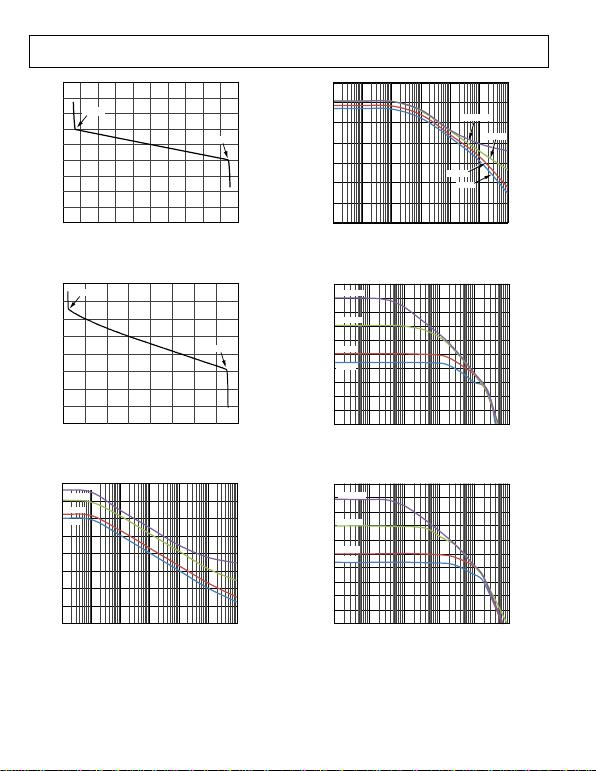Rev. 0 | Page 12 of 24
33
31
29
27
25
23
21
19
140
120
100
80
60
40
20
0
0.1 1 10 100 1k 10k 100k
FREQUENCY (Hz)
NEGATIVE PSRR (dB)
07759-024
17
15
–0.5 0 0.5 1.0 1.5 2.0 2.5 3.0 3.5 4.0 4.5
INPUT BIAS CURRENT (nA)
759-021
COMMON-MODE VOLTAGE (V)
07
–0.14V
+4.23V
Figure 21. Input Bias Current vs. Common-Mode Voltage, Vs = 5 V
40
35
30
25
20
15
10
5
0
–16 –12 –8 –4 0 4 8 12 16
INPUT BIAS CURRENT (nA)
9-022
COMMON-MODE VOLTAGE (V)
0775
–15.01V
+14.03V
Figure 22. Input Bias Current vs. Common-Mode Voltage, Vs = ±15 V
160
140
120
100
80
60
40
POSITIVE PSRR (dB)
20
0
0.1 1 10 100 1k 10k 100k
FREQUENCY (Hz)
07759-023
G = 1000
G = 100
G = 10
G = 5
Figure 23. Positive PSRR vs. Frequency, RTI
G = 5
G = 1000
G = 100
G = 10
Figure 24. Negative PSRR vs. Frequency
70
60
50
40
30
20
10
0
–10
–20
–30
100 1k 10k 100k 1M 10M
FREQUENCY (Hz)
GAIN (dB)
07759-025
G = 1000
G = 100
G = 10
G = 5
Figure 25. Gain vs. Frequency, VS = ±15 V
70
60
50
40
30
20
10
0
–10
–20
GAIN (dB)
–30
100 1k 10k 100k 1M 10M
FREQUENCY (Hz)
07759-026
G = 1000
G = 100
G = 10
G = 5
Figure 26. Gain vs. Frequency, VS = 2.7 V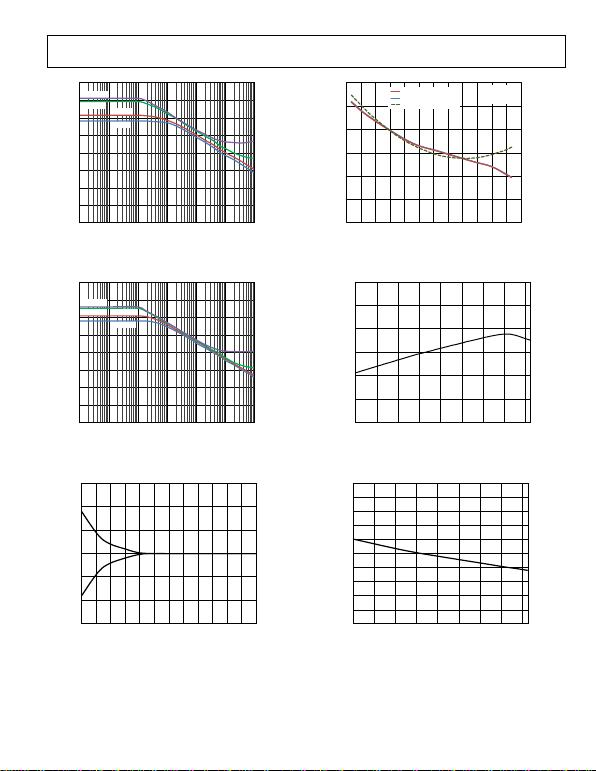Rev. 0 | Page 13
160
140
120
100
80
60
40
20
0
0.1 1 10 100 1k 10k 100k
CMRR (dB)
9-027
of 24
FREQUENCY (Hz)
0775
G = 1000
G = 100 G = 10
G = 5
35
30
25
20
15
10
150
125
100
75
50
25
0
5
–45 –30 –15 0 15 30 45 60 75 90 105 120 135
TEMPERATURE (°C)
INPUT BIAS CURRENT (nA)
INPUT OFFSET CURRENT (pA)
07759-030
V
S
= ±15V
V
REF
= 0V
–IN BIAS CURRENT
+IN BIAS CURRENT
OFFSET CURRENT
Figure 27. CMRR vs. Frequency, RTI
160
140
120
100
80
60
40
CMRR (dB)
Figure 30. Input Bias Current and Offset Current vs. Temperature
20
0
0.1 1 10 100 1k 10k 100k
759-028
FREQUENCY (Hz)
07
G = 1000
G = 100 G = 10
G = 5
Figure 28. CMRR vs. Frequency, RTI, 1 kΩ Source Imbalance
0.3
0.2
0.1
0
–0.1
–0.2
CHANGE IN INPUT OFFSET (µV)
300
200
100
0
–100
–200
–300
–40 –20 0 20 40 60 80 100 120
TEMPERATURE (°C)
GAIN ERROR (µV/V)
07759-031
Figure 31. Gain Error vs. Temperature, G = 5
10
8
6
4
2
0
–2
–4
–6
–8
CMRR (µV/V)
–10
–40 –20 0 20 40 60 80 100 120
TEMPERATURE (°C)
07759-032
Figure 32. CMRR vs. Temperature, G = 5
–0.3
0 10 20 30 40 50 60 70 80 90 100 110 120
WARM-UP TIME (s)
07759-029
Figure 29. Change in Input Offset Voltage vs. Warm-Up Time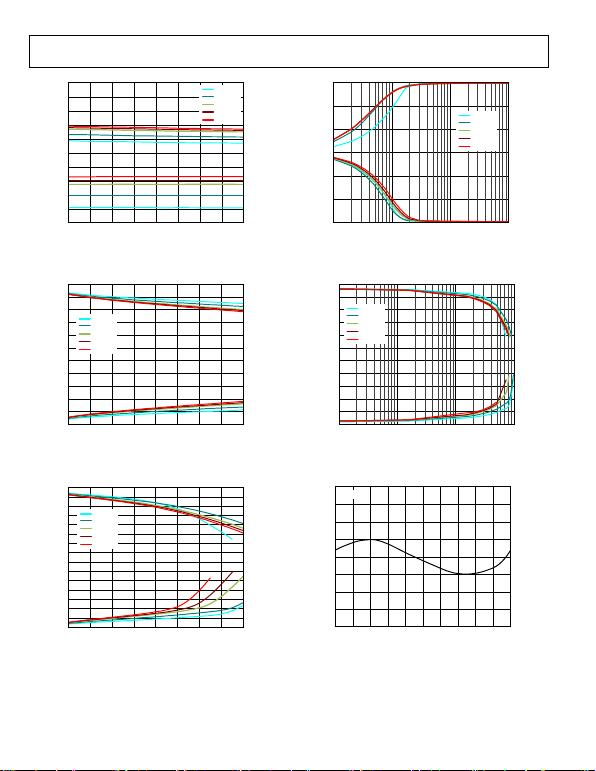Rev. 0 | Page 14 of 24
+V
S
–0.2
–0.4
–0.6
–0.8
–V
S
–0.2
–0.4
INPUT VOLTAGE (V)
REFERRED TO SUPPLY VOLTAGES
15
10
5
0
–5
–10
–15
100 1k 10k 100k
OUTPUT VOLTAGE SWING (V)
07759-036
–0.6
–0.8
2 4 6 8 10 12 14 16 18
759-033
SUPPLY VOLTAGE (±V
S
)
07
–40°C
+25°C
+85°C
+105°C
+125°C
Figure 33. Input Voltage Limit vs. Supply Voltage
+V
S
–0.1
–0.2
–0.3
–0.4
+0.4
+0.3
+0.2
–40°C
+25°C
+85°C
+105°C
+125°C
–V
S
+0.1
2 4 6 8 10 12 14 16 18
OUTPUT VOLTAGE SWING (V)
REFERRED TO SUPPLY VOLTAGES
759-034
SUPPLY VOLTAGE (±V
S
)
07
–40°C
+25°C
+85°C
+105°C
+125°C
Figure 34. Output Voltage Swing vs. Supply Voltage, RL = 10 kΩ
+V
S
–0.2
–0.4
+0.4
–0.6
+0.6
–0.8
+0.8
–1.0
+1.0
–1.2
+1.2
OUTPUT VOLTAGE SWING (V)
REFERRED TO SUPPLY VOLTAGES
Figure 36. Output Voltage Swing vs. Load Resistance
+V
S
–V
S
–0.2
–0.4
–0.6
–0.8
+0.8
+0.6
+0.4
+0.2
0.01 0.1 1 10
OUTPUT CURRENT (mA)
OUTPUT VOLTAGE SWING (V)
REFERRED TO SUPPLY VOLTAGES
07759-037
–40°C
+25°C
+85°C
+105°C
+125°C
Figure 37. Output Voltage Swing vs. Output Current
40
30
20
10
0
–10
–20
–30
NONLINEARITY (10ppm/DIV)
–40
–10 –8 –6 –4 –2 0 2 4 6 8 10
OUTPUT VOLTAGE (V)
07759-038
–V
S
+0.2
2 4 6 8 10 12 14 16 18
SUPPLY VOLTAGE (±V
S
)
07759-035
–40°C
+25°C
+85°C
+105°C
+125°C
Figure 35. Output Voltage Swing vs. Supply Voltage, RL = 2 kΩ
G = 5
Figure 38. Gain Nonlinearity, G = 5, RL ≥ 2 kΩ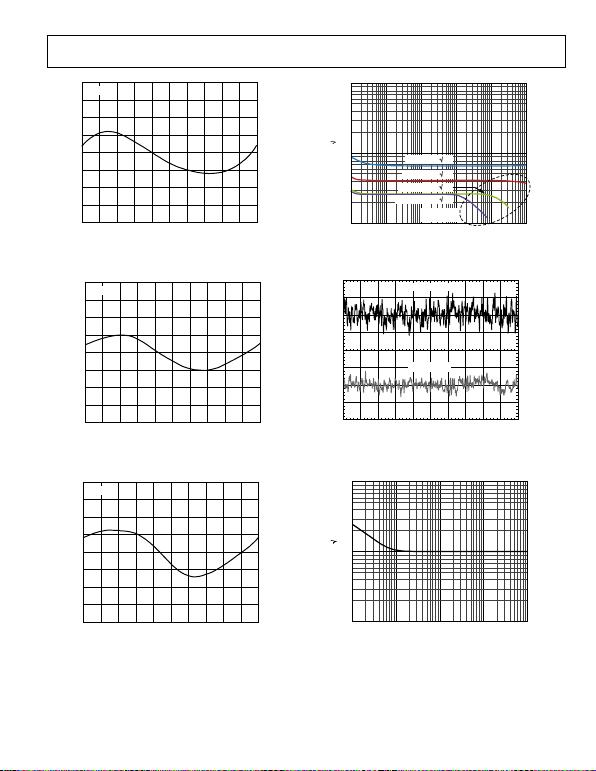Rev. 0 | Page 15 of 24
40
30
20
10
0
–10
–20
–30
–40
–10 –8 –6 –4 –2 0 2 4 6 8 10
NONLINEARITY (10ppm/DIV)
9-039
1k
100
10
1 10 100 1k 10k 100k
FREQUENCY (Hz)
NOISE (nV/
OUTPUT VOLTAGE (V)
0775
G = 10
Figure 39. Gain Nonlinearity, G = 10, RL ≥ 2 kΩ
160
120
80
40
0
–40
–80
–120
–160
108–6–42 0 2 4 6 8 10
NONLINEARITY (40ppm/DIV)
9-040
OUTPUT VOLTAGE (V)
0775
G = 100
Figure 40. Gain Nonlinearity, G = 100, RL ≥ 2 kΩ
400
300
200
100
0
–100
–200
NONLINEARITY (100ppm/DIV)
–300
–400
108–6–42 0 2 4 6 8 10
OUTPUT VOLTAGE (V)
07759-041
G = 1000
Figure 41. Gain Nonlinearity, G = 1000, RL ≥ 2 kΩ
Hz)
07759-042
G = 5 (67nV/ Hz)
G = 10 (40nV/ Hz)
G = 100 (26nV/ Hz)
G = 1000 (25nV/ Hz)
BANDWIDTH
LIMITED
Figure 42. Voltage Noise Spectral Density vs. Frequency
G = 1000, 200nV/DIV
G = 5, 1µV/DIV
7759-0430
Figure 43. 0.1 Hz to 10 Hz RTI Voltage Noise, G = 5, G = 1000
1k
100
NOISE (fA/
10
1 10 100 1k 10k
FREQUENCY (Hz)
07759-044
Figure 44. Current Noise Spectral Density vs. Frequency
Hz)